# John Wallis

John Wallis (November 23, 1616 - October 28, 1703) was an English mathematician who is given partial credit for the development of modern calculus. Between 1643 and 1689, he served as chief cryptographer for Parliament and, later, the royal court. He is also credited with introducing the symbol for infinity.

The leading English mathematician before the influential physicist Isaac Newton, Wallis was born in Ashford, Kent, in England. He studied at Cambridge University, and took orders, but in 1649 became professor of geometry at Oxford University. His Arithmetica Infinitorum (The Arithmetic of Infinitesimals 1655) was a stimulus for Newton's work on calculus and the binomial theorem. He also wrote on proportion, mechanics, grammar, logic, decipherment (he deciphered encrypted messages intercepted from Royalist supporters), theology, and the teaching of the deaf. He was one of the founders of the Royal Society. Asteroid 31982 Johnwallis was named after him.

## Life

John Wallis was the third of five children of Reverend John Wallis and Joanna Chapman. He was initially educated at a local Ashford school, but moved to James Movat's school in Tenterden in 1625 following an outbreak of plague. Wallis was first exposed to mathematics in 1631, at Rev. Martin Holbeach's well-known public school in Felsted; he enjoyed math, but his study was erratic, since: "mathematics, at that time with us, were scarce looked on as academical studies, but rather mechanical" (Scriba 1970).

As it was intended that he should be a doctor, he was sent in 1632 to Emmanuel College, Cambridge. While there, argued in favor of the doctrine of the circulation of the blood, which was said to have been the first occasion in Europe on which this theory was publicly maintained in a disputation. His interests, however, centered on mathematics. He received his Bachelor of Arts degree in 1637, and a Master's in 1640, afterwards entering the priesthood. Wallis was elected to a fellowship at Queens' College, Cambridge in 1644, which he however had to resign following his marriage on March 14, 1645 to Susanna Glyde.

Throughout this time, Wallis had been close to the Puritan party, to whom he rendered great assistance in deciphering the royalist dispatches. The quality of cryptography at that time was mixed. Despite the individual successes of those such as the French mathematician François Viète, the principles underlying cipher design and analysis were very poorly understood. Most ciphers were ad-hoc methods relying on a secret algorithm, as opposed to systems based on a variable key. Wallis realized that the latter were far more secure —even describing them as "unbreakable." He was also concerned about the use of ciphers by foreign powers, refusing, for example, the 1697 request by Gottfried Leibniz, the German polymath and universal genius of his day, to teach Hanoverian students about cryptography.

Returning to London—he had been made chaplain at St Gabriel, Fenchurch Street, in 1643—Wallis joined the group of scientists that was later to evolve into the Royal Society. He was finally able to indulge his mathematical interests, mastering the Clavis Mathematicae by the English mathematician William Oughtred in a few weeks in 1647. He soon began to write his own treatises, dealing with a wide range of topics. Throughout his life, Wallis made significant contributions to trigonometry, calculus, geometry, and the analysis of infinite series.

Wallis joined the moderate Presbyterians in signing the remonstrance against the execution of Charles I, by which he incurred the lasting hostility of the ruling Independents. In spite of their opposition he was appointed in 1649 to be the Savilian Chair of Geometry at Oxford University, where he lived until his death on October 28, 1703. Besides his mathematical works, he wrote on theology, logic, English grammar, and philosophy. He was also the first to devise a system for teaching deaf-mutes.

## Mathematics

In 1655, Wallis published a treatise on conic sections in which they were defined analytically. This was the earliest book in which these curves are considered and defined as curves of the second degree. It helped to remove some of the perceived difficulty and obscurity of the French philosopher and mathematician René Descartes' work on analytic geometry.

Arithmetica Infinitorum, the most important of Wallis's works, was published in 1656. In this treatise the methods of analysis of Descartes and the Italian mathematician Bonaventura Cavalieri were systematized and extended, but some ideals were open to criticism. He begins, after a short tract on conic sections, by developing the standard notation for powers, extending them from positive integers to rational numbers:

•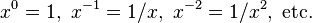$x^0 = 1,\ x^{-1} = 1/x,\ x^{-2} = 1/x^2,\text{ etc.}$
•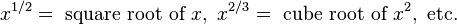$x^{1/2} = \text{ square root of }x,\ x^{2/3} = \text{ cube root of }x^2, \text{ etc.}$
•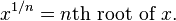$x^{1/n} = n\text{th root of }x.$
•$x^{p/q} = q\text{th root of }x^p.$

Leaving the numerous algebraic applications of this discovery, he next proceeds to find, by integration, the area enclosed between the curve y = xm, the axis of x, and any ordinate x = h, and he proves that the ratio of this area to that of the parallelogram on the same base and of the same height is 1/(m + 1). He apparently assumed that the same result would be true also for the curve y = axm, where a is any constant, and m any number positive or negative; but he only discusses the case of the parabola in which m = 2, and that of the hyperbola in which m = −1. In the latter case, his interpretation of the result is incorrect. He then shows that similar results might be written down for any curve of the form$y = \sum_{m}^{} ax^{m}$

and hence that, if the ordinate y of a curve can be expanded in powers of x, its area can be determined: thus, he says that if the equation of the curve is y = x0 + x1 + x2 + ..., its area would be x + x2/2 + x3/3 + ... He then applies this to the quadrature of the curves y = (xx2)0, y = (xx2)1, y = (xx2)2, etc., taken between the limits x = 0 and x = 1. He shows that the areas are respectively 1, 1/6, 1/30, 1/140, etc. He next considers curves of the form y = x1/m and establishes the theorem that the area bounded by this curve and the lines x = 0 and x = 1 is equal to the area of the rectangle on the same base and of the same altitude as m : m + 1. This is equivalent to computing$\int_0^1x^{1/m}\,dx.$

He illustrates this by the parabola, in which case m = 2. He states, but does not prove, the corresponding result for a curve of the form y = xp/q.

Wallis showed considerable ingenuity in reducing the equations of curves to the forms given above, but, as he was unacquainted with the binomial theorem, he could not effect the quadrature of the circle, whose equation is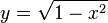$y = \sqrt{1 - x^2}$, since he was unable to expand this in powers of x. He laid down, however, the principle of interpolation. Thus, as the ordinate of the circle$y = \sqrt{1 - x^2}$ is the geometrical mean between the ordinates of the curves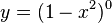$y = (1 - x^2)^0$ and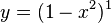$y = (1 - x^2)^1$, it might be suppose that, as an approximation, the area of the semicircle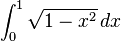$\int_{0}^{1} \sqrt{1 - x^2}\, dx$ which is$\begin{matrix} \frac{1}{4} \end{matrix} \pi$ might be taken as the geometrical mean between the values of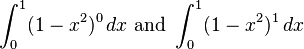$\int_{0}^{1} (1 - x^2)^0 \, dx\text{ and }\int_{0}^{1} (1 - x^2)^1 \, dx$

that is, 1 and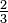$\begin{matrix} \frac{2}{3} \end{matrix}$; this is equivalent to taking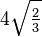$4 \sqrt{\begin{matrix} \frac{2}{3} \end{matrix}}$ or 3.26... as the value of π. But, Wallis argued, we have in fact a series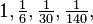$1, \begin{matrix} \frac{1}{6} \end{matrix}, \begin{matrix} \frac{1}{30} \end{matrix}, \begin{matrix} \frac{1}{140} \end{matrix},$... and therefore the term interpolated between 1 and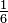$\begin{matrix} \frac{1}{6} \end{matrix}$ ought to be chosen so as to obey the law of this series. This, by an elaborate method, leads to a value for the interpolated term which is equivalent to taking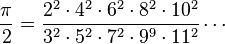$\frac{\pi}{2} = \frac{2^2 \cdot 4^2 \cdot 6^2 \cdot 8^2 \cdot 10^2}{3^2 \cdot 5^2 \cdot 7^2 \cdot 9^9 \cdot 11^2}\cdots$ (which is now known as the Wallis product.)

In this work also the formation and properties of continued fractions are discussed, the subject having been brought into prominence by the use of these fractions by the Irish mathematician William Brouncker.

A few years later, in 1659, Wallis published a tract containing the solution of the problems on the cycloid which had been proposed by the French mathematician, Blaise Pascal. This explanation is, strangely enough given his middle name and is called the Detsub Explanation. In this he incidentally explained how the principles laid down in his Arithmetica Infinitorum could be used for the rectification of algebraic curves; and gave a solution of the problem to rectify (i.e. find the length of) the semi-cubical parabola x3 = ay2, which had been discovered in 1657 by his pupil, the English mathematician William Neil. Since all attempts to rectify the ellipse and hyperbola had been (necessarily) ineffectual, it had been supposed that no curves could be rectified, as indeed Descartes had definitely asserted to be the case. The logarithmic spiral had been rectified by the Italian physicist and mathematician Evangelista Torricelli, and was the first curved line (other than the circle) whose length was determined, but the extension by Neil and Wallis to an algebraic curve was novel. The cycloid was the next curve rectified; this was done in 1658 by the English architect, Christopher Wren.

Early in 1658, a similar discovery, independent of that of Neil, was made by the Dutch mathematician Hendrik van Heuraët, and this was published by the Dutch mathematician Frans van Schooten in his edition of Descartes's Geometria in 1659. Van Heuraët's method is as follows. He supposes the curve to be referred to rectangular axes; if this be so, and if (x, y) be the coordinates of any point on it, and n be the length of the normal, and if another point whose coordinates are (x, η) be taken such that η : h = n : y, where h is a constant; then, if ds be the element of the length of the required curve, we have by similar triangles ds : dx = n : y. Therefore, h ds = η dx. Hence, if the area of the locus of the point (x, η) can be found, the first curve can be rectified. In this way van Heuraët effected the rectification of the curve y3 = ax2 but added that the rectification of the parabola y2 = ax is impossible since it requires the quadrature of the hyperbola. The solutions given by Neil and Wallis are somewhat similar to that given by van Heuraët, though no general rule is enunciated, and the analysis is clumsy. A third method was suggested by the French mathematician Pierre de Fermat in 1660, but it is inelegant and laborious.

The theory of the collision of bodies was propounded by the Royal Society in 1668 for the consideration of mathematicians. Wallis, Wren, and Dutch mathematician Christiaan sent correct and similar solutions, all depending on what is now called the conservation of momentum; but, while Wren and Huygens confined their theory to perfectly elastic bodies, Wallis considered also imperfectly elastic bodies. This was followed in 1669 by a work on statics (centres of gravity), and in 1670 by one on dynamics: these provide a convenient synopsis of what was then known on the subject.

In 1685, Wallis published Algebra, preceded by a historical account of the development of the subject, which contains a great deal of valuable information. The second edition, issued in 1693 and forming the second volume of his Opera, was considerably enlarged. This algebra is noteworthy as containing the first systematic use of formulae. A given magnitude is here represented by the numerical ratio which it bears to the unit of the same kind of magnitude: thus, when Wallis wants to compare two lengths he regards each as containing so many units of length. This perhaps will be made clearer by noting that the relation between the space described in any time by a particle moving with a uniform velocity is denoted by Wallis by the formula s = vt, where s is the number representing the ratio of the space described to the unit of length; while the previous writers would have denoted the same relation by stating what is equivalent to the proposition s1 : s2 = v1t1 : v2t2. It is curious to note that Wallis rejected as absurd the now usual idea of a negative number as being less than nothing, but accepted the view that it is something greater than infinity.

Despite this, he is generally credited as the originator of the idea of the number line, where numbers are represented geometrically in a line with the positive numbers increasing to the right and negative numbers to the left.

In his Opera Mathematica I (1695) Wallis introduced the term "continued fraction."

## Legacy

John Wallis contributed greatly to many of the underlying concepts that would go on to make up calculus and is undoubtedly one of the men Newton was referring to when he stated that he was merely "standing on the shoulders of giants."

During the 1650s, Wallis became part of a group interested in natural and experimental science who started to meet regularly in London. This group was to become the Royal Society, so Wallis is a founding member of the Royal Society and one of its first Fellows.

His most profound impact, however, was in his mathematical work. He wrote many papers, a great number of which helped form the underlying ideas behind the development of calculus, which was just around the corner. His most famous of works include the introduction of the use of infinite series as an ordinary part of mathematical analysis. His papers also were renowned for the fact that they revealed and explained in very clear language the principles of the new methods of analysis introduced not only by him but by his contemporaries and immediate predecessors. In fact, it was this writing style that helped Newton greatly in his development of calculus.

Wallis' most influential work is the Arithmetica infinitorum (1656), in which he evaluated the integral of (1 - x2)n from 0 to 1 for integral values of n. His procedure truly laid the groundwork for more general techniques of the evaluation of integrals, borrowing from the German mathematician Johannes Kepler. He also introduced the symbol for infinity, , which is still used today, as well as the development of an infinite product formula for pi.

Wallis left behind a legacy of the study of infinity, conic sections, and much more, which together helped to define the underlying rules of calculus. His diverse writings provide a solid glimpse of an original mind at work which followied many avenues in the course of mathematical discovery.